﻿ Complete Design and Assemble of Wind Turbine BaldPublications are Open
Access in this journal
Article Versions
Export Article
• Normal Style
• MLA Style
• APA Style
• Chicago Style
Review Article
Open Access Peer-reviewed

### Complete Design and Assemble of Wind Turbine Bald

Mohammed Y. Abdellah , Mohammad Sh. Alazemi, Ahmed F. Mohamed, G. Tag Abdel-Jaber
American Journal of Mechanical Engineering. 2019, 7(1), 9-19. DOI: 10.12691/ajme-7-1-2
Received December 26, 2018; Revised January 01, 2019; Accepted February 09, 2019

### Abstract

the feasibilities of using wind energy in the Kuwait have analyzed and investigated. The wind speed level and resources in Kuwait was investigated experimentally. The aerodynamic of the rotor of wind turbine is investigated experimentally. Aerodynamic of wind turbines is studied theoretical using Flo software based on the theoretical models. All components of wind turbine are selected and manufactured in Kuwait. The required materials for assembling rotor and main shaft were performed. The material of the turbine is investigated for stage of building and assembling.

### 1. Introduction

Today, the cost per kWh energy generated by the wind turbine is reduced to half of the cast 10 years ago. It is provided that this development will continue and it is generally expected that the wind power technology will competitive other types of energy sources such as solar energy, thermal energy, etc 1. Photo shown in Figure 1, shows the biggest turbine which installed in the Brittin, it capacity is 10MW. There are two methods of using wind energy in the generation electricity. Beedell et al. 2, studied methods of connection wind turbine technology with general applications. The common method is related to grid-connected wind turbines. This method is considered commercially available and mature. Also, this method is simple and not complicated. The overall cost of theses turbines is low. A lot of wind turbines are installing in different places from world such as USA, Demark, Holanda, Germany, Egypt, etc.

• Figure 1. Modern biggest wind turbine 10 MW install in Britain 

The other method of connection wind turbine is standalone station. This method can be used in the very remote area and there is not public electrical grid there. The cost of this method is high and very complicated. Also, there are a lot of troubles and problems can be occurred during operation of theses turbines. In the Middle East, a lot of windmills were installed throughout by the 20 century and were installed in the farms and groups. The Dutch refined the windmill and adapted it to other uses, particularly, draining lakes and marshes in the Rhine River Delta. Through 1900s, small wind systems were developed to generate DC current that was stored in a bank of batteries. Most of these units was used in alone station and used rural areas where as the connection of grid power is very expensive, Development of large electrical windmills begins in Denmark as early as 1890, though most other countries failed to initiate efforts until the 1930s, 4. The most successful commercial wind turbines as of now are machines of installed capacities of up to 2.0 MW, and at this time the next generation on turbines in the 3 MW size are being tested. It is known that turbine availability is its ability to work continually when wind speed is higher than start up limit. In most designs the technical availability of turbines is reached 98-99 % approximately in Beedell et al. 4 in year 2014, India has been installed very large wind farm. The total capacity of this wind is reached 28500 MW. This farm is considered from the fifth largest wind farm in the world. The minimum capacity of turbine is 5 MW. All turbine from types of horizontal axis wind turbines. The percentage of wind power in India reached nearly 8.5% of total installed power generation capacity 5, 6, 7, 8, 9, 10, 11, 12, 13.

Many works 14, 15, 16, 17, 18, 19 investigated the feasibility of wind turbines in KIA, Jal Aliyah, Airport, Al-Salmi, Al-wafra, and Abdaly etc. The meteorological data were used to evaluate and analyze wind energy characteristics and to determine the location with highest wind energy potential. Their studied established ability of wind energy but all study was on feasibility study, the electricity amount not consider in their study.

The main goal of the present study is to give a complete design and assembly to the Wind Turbine bald, also give a method of measuring energy then simulated using Flo software.

### 2. Design of Wind Turbine Bald

Equation 1 can be used to determine the wind turbine tip speed ratio. Tip speed ratio is defined as the ration of tip speed of blade to relative wind speed. This equation can be written as following: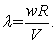(1)

In equation 1, λ is the tip speed ratio, ω is the rotor rotational speed in radians per second, R is the rotor radius in meters and V is the wind speed.

• Figure 2. Power Turbine tip Speedy ration with power coefficient 
2.1. Design Assumptions

Power rated = 500 w, Power coefficient = 0.1- 0.15, design wind speed = 12 m/s, Air density = 1.25 kg/m3, No. of Magnus Turbine cylinder used = 3 and No. of Savonius Turbine blades used = 3.

The blade for turbine consists of two parts Savonius and Magnus. To select the size of blade first assume the cylinder diameter for Magnus part is, d= 0.16 m.

• Figure 3. Blade style of suggest turbine
2.3. Aerodynamic Forces Effect on Turbine

Drag force on Savonius part, FD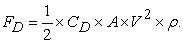(2)

Lift force on cylinder part, FL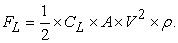(3)

The rotor Power output is given by: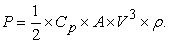(4)

CD= drag coefficient

CL: lift force coefficient

A: area of rotor, m2

V: wind speed, m/s

ρ: air density.

2.4. Rotor Design

The first step is design rotor blades and cylinder part. The required power is 500 W.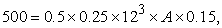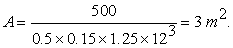A = total area of rotor = DH

Assuming D = 2.4 m

H = A/D = 3/2.4= 1.25 m

Where,

D: diameter of rotor

H: height of

2.5. Savonius Gap (e)

Different investigation studied the opium value of air gap in Savonius rotor blade. 21 recommended that the opium value of e can be taken as eopt = d/6. This value will give the best aerodynamic forces on the Savonius rotor (see Figure 4).

b+ b-e = 6, e = b/6 for optimum 16.

2b – e/b = d

1.83 b = 0.16

b =0.16/1.83 = 0.09m = 9 cm

then e= 9/6= 1.5cm

• Figure 4. Definition of the values d, b and e for Savonius rotor

Take the length of Savonius blade part 25% from total height Ls = 0.25 × 1.25= 0.31 m, Then the length of Magnus blade LM= 1.25- 0.31= 0.94 m, Then the total height = H= 0.31+0.94= 1.25m.

2.6. Rotor Solidity

The solidity of a wind turbine rotor is the ratio between the area of the blades projected into the rotor plane and the total area covered by the rotating blades, Ablade- The combined solidity, i.e. the sum of solidities of the blades, is hence: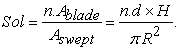Where n is the number of wind turbine blades, 3. Where R is rotor radius, R= D/2= 2.4/2= 1.2 m.

A blade = cylinder surface area = π ×x d × H= 3.14×0.16×1.25= 0.628 m2, A swept = πxR2= 3.14×2.42= 18 m2.

Then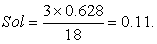Acceptable value for most wind turbines.

2.7. Torque Calculations

From Diagram given in Figure 5 it can select proper tip speed ratio for turbine as λ=2 approx.

λ= 2 = ωR/V, then R= rotor radius = 1.2 m, V= 12 m/s, ω: angular velocity of rotor at design wind speed, Then, ω= λ × V/R= 2×12/1.2=20 rad/s.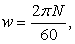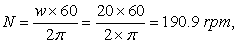take the rotor speed = 200 rpm, and bn Power = Torque x angular velocity.

P = T× ω.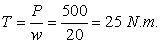Where, T is transmitted torque by rotor of turbine. Another equation is used in design of wind turbine shaft.

2.8. Selecting Turbine Generator

The maximum generator shaft torque can be calculated using equation 22: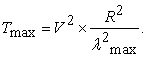Take wind speed maximum, Vmax = 25 m/s.

R= rotor radius = 1.2 m, λ max = 4 for Magnus/Savonius turbines, T max= 252x 1.22/42 = 56.25 Nm. As discussed in previous chapter that the generator type is Permanent DC, 12/24 v. the rated power is 500 W. The maximum Tip speed at speed V= 25m/s is increased to highest value of 4. The power output from generator is 500 W at wind speed of 12 m/s and at 200 rpm. Take charged voltage for generator is 16 v, then Generator ampere I = Power/voltage = 500/16= 31.25 A.

2.9. Battery Specification

Voltage: 12 V, Ampere: 42 A, Battery active power = VxI = 42x12= 504W.

2.10. Main Shaft Design

The shaft should be design as the following loading (see Figure 5):

1. shear stress due torque on the shaft

2. bending moment due to axial thrust on the shaft from wind

3. fatigue due to cycling load

• Figure 5. turbine aerodynamic & parts

Axial thrust force calculation:

Assume, Fao = Maximum (theoretical) axial thrust on the horizontal axis machine (see Figure 6).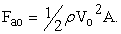But the actual thrust is the rate of momentum of the airstreams on disk, then: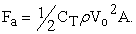If CT is the thrust coefficient, which defined as the fraction of thrust force experienced by the actual turbine. In practice the value of CT on the wind turbine appears to the wind as = (0.2-0.45). From power curve given in Appendix C, the rated power occurs at wind speed of 25 m/s, so take, CT = 0.25, Vo = 25 m/s, ρ = 1.25 kg/m3, A of one blade= 3m2 from rotor design.

Then,

Fa = 0.5 × 0.25 × 1.25 × 252 × 3 = 292.96= 293 N, in most design of vertical turbine, the total shaft length.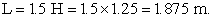With assuming the thrust force effect on the midpoint of shaft horizontally, then Bending moment = M max = W×L/4 = 293x1.875/4= 137.3 Nm.

By using ASME code equation for shaft design we have, 23.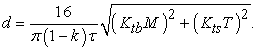Assume:

For 15 reduction in area of shaft due to fixing rotor and generator using keyways, then k=0.15, from Tables, for rotating shaft with minor shock loads, Ktb = 1.5 and Kts = 1. Assume the shaft is made of 1018, cold rolled steel, from Table A-20, Sut = 440 MPa, Syt= 370 MPa, and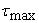= 370/2 = 185 MPa, taking factor of safety n, then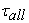= 185/2 = 92.5 MPa.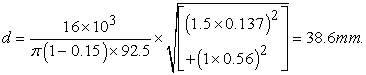Take the shaft design as 40mmfor safety see (Figure 7).

• Figure 7. shaft drawing & dimensions

Because there is thrust at bearing B, use Table 11-2 to select bearing size as following: For angular –contact ball bearing, it can select bearing as series – 02: take Bore = 35 mm, OD = 72 mm, width 17 mm (see Figure 8).

• Figure 8. Bearing dimensions
2.11. Braking System Design

The braking system consists of hydraulic pump, solenoid valve, oil tank caliper, disc with pads (see Figure 9). In most design of wind turbine, the braking torque = 2-3 maximum torque on the rotor. At wind speed 25 m/s, the maximum rotor torque is 56.25 Nm. So, the brake torque can be estimated as following: Tb= 2 ×Tmax= 2×56.25= 112.5 Nm, Assume the dice selected diameter is 15 cm, then Dics radius = 0.15/2= 0.075 m, Brake force = Fb = T/r= 112.5/0.075= 1500 N 23.

• Figure 9. braking parts on the turbine

Hydraulic pressure needed p= F/AP, Where AP is piston area of hydraulic pump, for 20 mm diameter of pump piston, dp= 0.02m, Ap= (3.14/4) ×0.022= 3.14×10-4 m2, Pressure p= 1500/3.14×10-4=4.777 MPa.

2.12. Suggested Raw Material & Accessories of Proposed Wind Turbine

Rotor material:

Figure 10 shows the main parts of suggested turbine which will be build in the second stage from the project.

The rotor consists of cylinder, Savonius blade, shaft, bearing. The cylinder will make from plastic containers with diameter 0.16 m and suitable thickness. The Savonius part will make also, from plastic sheets or old containers with width 9 cm and gap 1.5 cm and total height is 31 cm. This rotor will use Savonius and Magnus effect in the consideration. The Savonius rotor of turbine will be designed with a set of blades; it can use also plastic PVC tubes with suitable diameter for manufacturing blade and Magnus cylinder. In this case, it can get on the low cost, and symmetrical shape of design prototype. It can use pipe with outer diameter of 6.5 inch (16 cm). The 6.5 inch PVC pipe will be cut to the sizes specified and glued together using PVC cement. The buckets were cut into 2” half cylinder ribs and attached to the PVC frame. The rib structure was then covered in one layer of chicken wire to add stability while adding very little weight. To connect the blades to the axle of the bike rims, a flange nut can be epoxy into a PVC bushing which was glued into the blade assembly.

2.13. Shaft

The main shaft can select from mild steel, with main diameter of 40 mm with length 1.9 m approximately. For decreasing the weight, it can use aluminum shaft or hallow iron shaft (see Figure 11).

• Figure 10. Flow chart of proposed turbine
2.14. Bearing

Two ball bearing can be selected for decreasing friction on the main shaft of turbine. The ball bearing can be decreasing the operating starting required torque of turbine as shown in Figure 12.

• Figure 12. Ball bearing different components

The inner diameter is 35 mm, outer diameter is 72 mm. So, the overall parts needed for manufacturing rotor can be summarized as:

1. PVC pipe, sheet plastic

2. 2 ball Bearings (bottom one needs to be able to handle a load)

3. Grease nipple

4. A rod 40 mm round aluminum for shaft

5. wood

6. sheet metal with thickness 2 cm

2.15. Turbine Tower

The tower was designed to be 10 m in height to give efficient wind speed. It will use old scraps from wood or iron tube for assembling the tower. The tower will be made as rectangular cross-sectional area with 50x50 cm. two iron plate or sheet with thickness 10 mm was used to fix generator with building and generator with tower.

2.16. Selected Generator Specifications

Permanent Magnet DC variable speed generator will be used when assembling the turbine. There are different types and styles on the market that can used with wind turbines. Several generators can be used to capable of providing a decent power at rotational speeds well below those required for a wind turbine.

− Power rated 500 W at 200 rpm

− DC output

− Output voltage 12/24 v

− Amps. 35 A

− Variable speed

− Can be used with vertical turbines

− No. of phase is one

− No. of poles 4

− Self excited

− Gearless

### 3. Wind Turbine Blade Test

After compiling background research, we analyzed the information and decided upon two chosen turbine blade designs that could be tested to demonstrate improved results. The two designs were an adjustable angle S1223 airfoil design and a split Savonius airfoil design. To begin the design process, we kept the some of the parameters of the old design, such as turbine height, blade size, and diameter of the VAWT. An additional design parameter was to allow each blade to rotate 360° and provide them with a capability to be locked at any desired angle. The airfoil design was also comprised of three blades because research indicates 3 blades for wind turbines with airfoils is ideal for limiting vibrations and increasing efficiency (see Figure 13).

• Figure 13. rotor design configuration

Prior to designing the split Savonius wind turbine, we created parameters in order to allow testing to be assessed in comparison to testing in previous projects. These parameters assured that the turbine blades were of same height, and the total radii of the blades are the same as the flat bladed designs from this study.

After examining different wind turbines, we decided that a simple split Savonius design would fit best for our application. The split Savonius we designed had a zero offset indicating that where the overlapped cusps meet the distance in the y-axis is zero. This can be seen in Figure 13, 3 where the red line indicates the zero offset. In addition, the overlapping cusps had an offset of 1.5 inches in order to surpass the 0.5-inch rod, located in the center of the blades, without creating interference and allow for wind to pass past the rod (see Figure 14).

• Figure 14. shows Savonius blade size

### 4. Assemble of Wind Turbine Bald

The Savonius rotor of turbine is designed with a set of blades. Metal sheet was used to manufacture the blades of Savonius and Magnus turbine rotor. The thickness of sheet is 0.4 mm. also bicycle two tiers was used to assembling the rotor of the turbine. In this case, it can get on the low cost, and symmetrical shape of design prototype. It can use pipe with outer diameter of 6.5 inch (16cm). The blades were designed and drawer on the metal plate sheet. Then the blade was cutting and round to the required shape. The sheet metal was assembled into 2” half cylinder ribs and attached to the bicycle frame. The rib structure was then covered in one layer of wire to add stability while adding very little weight. To connect the blades to the axle of the bike rims, a flange nut can be epoxied into a frame bushing which was glued into the blade assembly (see Figure 15).

• Figure 15. final blade shape

### 5. Turbine Tower

The tower was designed to be 3.0 m in height to give efficient wind speed. It will use old scraps from wood or iron tube for assembling the tower. The tower will be made as rectangular cross-sectional area with 50x50 cm. two iron plate or sheet with thickness 10 mm was used to fix generator with building and generator with tower as shown in Figure 16.

• Figure 16. Turbine tower
5.1. Selected Generator

There are different types and styles on the market that can used with wind turbines. Several generators can be used to capable of providing a decent power at rotational speeds well below those required for a wind turbine.

Main specifications:

− Alternator Spec., Car Kia

− Power rated 500 W at 200 rpm

− DC output

− Output voltage 12/24 v

− Amp =50

− Can be used with vertical turbines

− No. of phase is one

− No. of poles 4

− Self excited

### 6. Flo-Program Results

Check size (94cm Magnus / 31cm Savonius), In this part the rotor of turbine was tested and simulated by using Flo-software. The results of program was tested and compared with other results (see Figure 17, Figure 18, Figure 19, Figure 20, Figure 21, Figure 22, Figure 23 and Figure 24) and Table 1 and Table 2.

• Figure 17. blade test (v=5m/s)
• Figure 18. drag coefficient with wind speed 10 m/s
• Figure 19. drag coefficient with different size of blades
• Figure 20. drag coefficient of turbine (20m/s)
• Figure 21. Drag coefficient at wind speed 10m/s
• Figure 22. drag coefficient comparison with standard values at 5 m/s wind speed
• Figure 23. drag coefficient comparison with standard values at 20 m/s wind speed
• Figure 24. drag coefficient comparison with standard values at 10 m/s wind speed

### 7. Conclusion

The use of alternative energy in Kuwait is important for three reasons: the growing demand for electricity, the high price of oil and the optimal environment for investing in alternative energy as Kuwait is abundant with bright sun and wind. Kuwait attempted its first Renewable Energy Programme in 1975-1988 by installing a number of solar facilities which were neglected when the programme was abandoned due to the expense of materials and operations. it was concentrated on the wind measured and resources level in Kuwait. The wind variability through period 1980 to 2014 was analyzed. The capacity factor for applying commercial wind turbine in Kuwait was calculated. Also, the facilities of using wind energy in Kuwait was covered. Effect of wind turbines on the avian, noise, grid, land, etc. were analyzed.

### References

  E. W. Golding, generation of electricity by wind power: Spon, 1976. In article  P. A. Vine, London's lost route to the sea: an historical account of the inland navigations which linked the Thames to the English Channel: David & Charles Publishers, 1986. In article PubMed  R. Pena, J. Clare, and G. Asher, "Doubly fed induction generator using back-to-back PWM converters and its application to variable-speed wind-energy generation," IEE Proceedings-Electric power applications, vol. 143, pp. 231-241, 1996. In article View Article  J. Beedell and T. Rehman, "Explaining farmers' conservation behaviour: Why do farmers behave the way they do?," Journal of Environmental management, vol. 57, pp. 165-176, 1999. In article View Article  Y. M. Mohamed K. Hassan, Abu El-Ainin H, "Improvement of Al-6061 alloys mechanical properties by controlling processing parameters," International Journal of Mechanical & Mechatronics Engineering IJMME-IJENS, vol. 12, pp. 14-18, 2012. In article  Y. Mohammed, M. K. Hassan, and A. Hashem, "Analytical model to predict multiaxial laminate fracture toughness from 0 ply fracture toughness," Polymer Engineering & Science, vol. 54, pp. 234-238, 2014. In article View Article  Y. Mohammed, M. K. Hassan, and A. Hashem, "Effect of stacking sequence and geometric scaling on the brittleness number of glass fiber composite laminate with stress raiser," Science and Engineering of Composite Materials, vol. 21, pp. 281-288, 2014. In article View Article  M. Y. Abdellah, M. S. Alsoufi, M. K. Hassan, H. A. Ghulman, and A. F. Mohamed, "Extended finite element numerical analysis of scale effect in notched glass fiber reinforced epoxy composite," Archive of Mechanical Engineering, vol. 62, pp. 217-236, 2015. In article View Article  K. H. Mohamed, Y. A. Mohammed, S. Azabi, K., and W. W. Marzouk, "Investigation of the Mechanical Behavior of Novel Fiber Metal Laminates," International Journal of Mechanical & Mechatronics Engineering IJMME-IJENS, vol. 15, pp. 112-118, 2015. In article  M. Y. Abdellah, "Delamination Modeling of Double Cantilever Beam of Unidirectional Composite Laminates," Failure analysis and prevention, 2017. In article  M. Y. A. Ayman M. M. Abdelhaleem, Hesham I. Fathi, Montasser Dewidar, "Mechanical Properties of ABS Embedded with Basalt Fiber Fillers," Journal for Manufacturing Science and Production, 2016. In article  M. K. Hassan, M. Y. Abdellah, S. K. Azabi, and W. Marzouk, "Fracture Toughness of a Novel GLARE Composite Material," 2015. In article  N. S. Korim, M. Y. Abdellah, M. Dewidar, and A. M. Abdelhaleem, "Crushable Finite Element Modeling of Mechanical Properties of Titanium Foam," 2015. In article  S. Mathew, Wind energy: fundamentals, resource analysis and economics vol. 1: Springer, 2006. In article View Article  PubMed  A. Hajiah, T. Khatib, K. Sopian, and M. Sebzali, "Performance of grid-connected photovoltaic system in two sites in Kuwait," International Journal of Photoenergy, vol. 2012, 2012. In article  A. Hajiah and M. Sebzali, "Optimal sizing of wind power systems in three high wind potential zones in Kuwait for remote housing electrification," International Journal of Renewable Energy Research (IJRER), vol. 3, pp. 167-171, 2013. In article  A. Hajiah, M. Sebzali, and S. Ebrahim, "Analysis of wind power potential characteristics and feasibility in Kuwait for possible electricity production," International Journal of Renewable Energy Research (IJRER), vol. 3, pp. 812-818, 2013. In article  A. Hansen and C. Butterfield, "Aerodynamics of horizontal-axis wind turbines," Annual Review of Fluid Mechanics, vol. 25, pp. 115-149, 1993. In article View Article  P. Higgins and A. Foley, "The evolution of offshore wind power in the United Kingdom," Renewable and sustainable energy reviews, vol. 37, pp. 599-612, 2014. In article View Article  W. Z. Shen, M. O. Hansen, and J. N. Sørensen, "Determination of angle of attack (AOA) for rotating blades," in Wind Energy, ed: Springer, 2007, pp. 205-209. In article View Article  K. Bullis, "How Tesla is driving electric car innovation," MIT Technol. Rev., 2013. In article  N. Goudarzi and W. Zhu, "A review on the development of wind turbine generators across the world," International Journal of Dynamics and Control, vol. 1, pp. 192-202, 2013. In article View Article  R. G. Budynas and J. K. Nisbett, Shigley's mechanical engineering design vol. 8: McGraw-Hill New York, 2008. In article

Published with license by Science and Education Publishing, Copyright © 2019 Mohammed Y. Abdellah, Mohammad Sh. Alazemi, Ahmed F. Mohamed and G. Tag Abdel-JaberThis work is licensed under a Creative Commons Attribution 4.0 International License. To view a copy of this license, visit http://creativecommons.org/licenses/by/4.0/